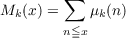#### Vol. 32, No. 1, 1970

 Download this articleFor screen For printingRecent Issues Vol. 325: 1  2 Vol. 324: 1  2 Vol. 323: 1  2 Vol. 322: 1  2 Vol. 321: 1  2 Vol. 320: 1  2 Vol. 319: 1  2 Vol. 318: 1  2Online Archive Volume: Issue:The Journal Subscriptions Editorial Board Officers Contacts Submission Guidelines Submission Form Policies for Authors ISSN: 1945-5844 (e-only) ISSN: 0030-8730 (print) Special Issues Author Index To Appear Other MSP Journals
Möbius functions of order k

### Tom M. (Mike) Apostol

Vol. 32 (1970), No. 1, 21–27
##### Abstract

Let k denote a fixed positive integer. We define an arithmetical function μk, the Möbius function of order k, as follows:

 μk(1) = 1, μk(n) = 0 if pk+1|n for some prime p, μk(n) = (−1)r if n = p 1k⋯p rk ∏ i>rpiai ,0 ≦ ai < k, μk(n) = 1 otherwise.
In other words, μk(n) vanishes if n is divisible by the (k + 1)-st power of some prime; otherwise, μk(n) is 1 unless the prime factorization of n contains the k-th powers of exactly r distinct primes, in which case μk(n) = (1)r. When k = 1k(n) is the usual Möbius function, μ1(n) = μ(n).

This paper discusses some of the relations that hold among the functions μk for various values of k. We use these to derive an asymptotic formula for the summatory functionfor each k 2. Unfortunately, the analysis sheds no light on the behavior of the function M1(x) = nxμ(n).

Primary: 10.43
##### Milestones